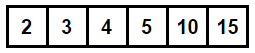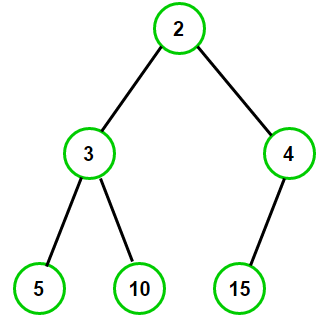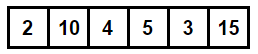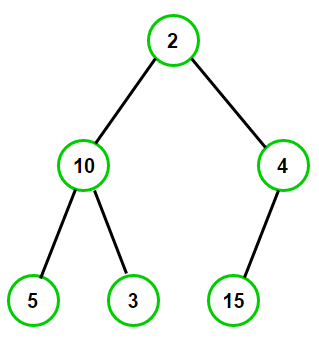# Check if an array represents min heap or not

Given an array of integers, check if it represents min heap or not.

For example, the first array represents a min heap but the second array don’t, as it violates the heap property.Since the input is an array, structural property of heap (all levels except the last level are full) cannot be violated. We only have to worry about the Min-Heap Property (priority of a node is at least as large as that of its parent) for given problem.

Since array indexing begins from 0, for a given array index i, if (2*i + 2 > n) is true then A[i] represents a leaf node. Otherwise when (2*i + 2 <= n) is true, A[i] represents internal heap node. Here, n is the size of the array.

For instance, consider array [2, 3, 4, 5, 10, 15] of size n = 6. Here [2, 3, 4] are internal nodes as (2*i + 2 <= 6) is true and [5, 10, 15] are leaf nodes as (2*i + 2 > 6) is true.Now that we know how to differentiate between a leaf node and a internal node based on given array index and number of nodes, lets come back to the given problem.

We can efficiently solve this problem by using recursion. The idea is to start from the root node (at index 0) and check if left and right child (if any) of root node is greater than the root node or not. If yes, we recursively call the procedure for both its children else we return false from the function. Below is the complete algorithm -

• If current node is a leaf node, return true as every leaf node is a heap
• If current node is an internal node,
• Recursively check if left child is min-heap or not
• Recursively check if right child is min-heap or not (if it exists)
• Return true if both left and right child are min-heap, else return false.

## Java

Output:

Given array is a min heap

#### Iterative C++ and Java implementation –

As recursion is costly, we can easily convert above recursive function to iterative one -

## Java

Output:

Given array is a min heap

The time complexity of above solutions is O(n) and auxiliary space used by the code is O(1).

Exercise: Given an array of integers, check if it represents Max-Heap or not.(1 votes, average: 5.00 out of 5)Loading...

Please use our online compiler to post code in comments. To contribute, get in touch with us.
Like us? Please spread the word and help us grow. Happy coding 🙂